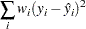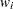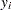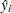WEIGHT Statement
WEIGHT variable ;

When a WEIGHT statement is specified, a weighted residual sum of squaresis minimized, whereis the value of the variable specified in the WEIGHT statement,is the observed value of the response variable, andis the predicted value of the response variable.

The observation is used in the analysis only if the value of the WEIGHT statement variable is greater than zero. The WEIGHT statement has no effect on degrees of freedom or number of observations. If the weights for the observations are proportional to the reciprocals of the error variances, then the weighted least squares estimates are best linear unbiased estimators (BLUE).